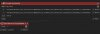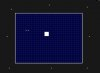# Drag And DropSpawning Enemies at random locations outside room

V

#### Vignesh Krishnan

##### Guest
Hey guys, so I am using Create Instance to create an Enemy Instance. I've set the X to be chosen between the X co-ordinate of 8 different instances of a spawner object. I've done the same for Y.

Now the problem is, enemy instances are sometimes created inside the room since it chooses X and Y co-ordinates separately. Which causes some of the positions to be right in the middle of the room.

Is there any way to avoid instances being created inside the room?Above is what I have used to create instancesThe empty objects outside the room are the spawn locations whose X and Y co-ordinates I've set

#### Jakylgamer

##### Member
well i know you stated drag and drop but to be honest i think this would be a lot easier with some simple code
create event: (controller spawn object)
Code:
``````//point index is the spawn position(think of it like an object placed in the room)
point_index = 0
spawn_positions = 1; // number of span positions (0 is a position)
//we set up a little 2d array
//spawn point 1
spawn_point[0,0] = -32;                        // x position if first spawn point
spawn_point[0,1] = 32;                         // y position of first spawn point
//spawn point 2
spawn_point[1,0] = (room_width+32);            // x position if second spawn point
spawn_point[1,1] = 32;                         // y position of second spawn point
//etc...``````
then all you would need to do to spawn the instances is choose a random spawn_point
in an alarm event
Code:
``````point_index = irandom(spawn_positions); //choose a random spawn point each time you spawn an enemy
instance_create_layer(spawn_point[point_index,0],spawn_point[point_index,1],"Enemy",obj_enemy)``````
this may seem like a lot so i made an example of it
https://puu.sh/BzVaj/f611d39776.yyz

Last edited:
V

#### Vignesh Krishnan

##### Guest
well i know you stated drag and drop but to be honest i think this would be a lot easier with some simple code
create event: (controller spawn object)
Code:
``````//point index is the spawn position(think of it like an object placed in the room)
point_index = 0
spawn_positions = 1; // number of span positions (0 is a position)
//we set up a little 2d array
//spawn point 1
spawn_point[0,0] = -32;                        // x position if first spawn point
spawn_point[0,1] = 32;                         // y position of first spawn point
//spawn point 2
spawn_point[1,0] = (room_width+32);            // x position if second spawn point
spawn_point[1,1] = 32;                         // y position of second spawn point
//etc...``````
then all you would need to do to spawn the instances is choose a random spawn_point
in an alarm event
Code:
``````point_index = irandom(spawn_positions); //choose a random spawn point each time you spawn an enemy
instance_create_layer(spawn_point[point_index,0],spawn_point[point_index,1],"Enemy",obj_enemy)``````
this may seem like a lot so i made an example of it
https://puu.sh/BzVaj/f611d39776.yyz
Thanks a lot! That's what I needed.

#### TheouAegis

##### Member
The problem with your original cose was you randomized x and y. You needed to pick an instance at random, then set x and y to that instance's x and y